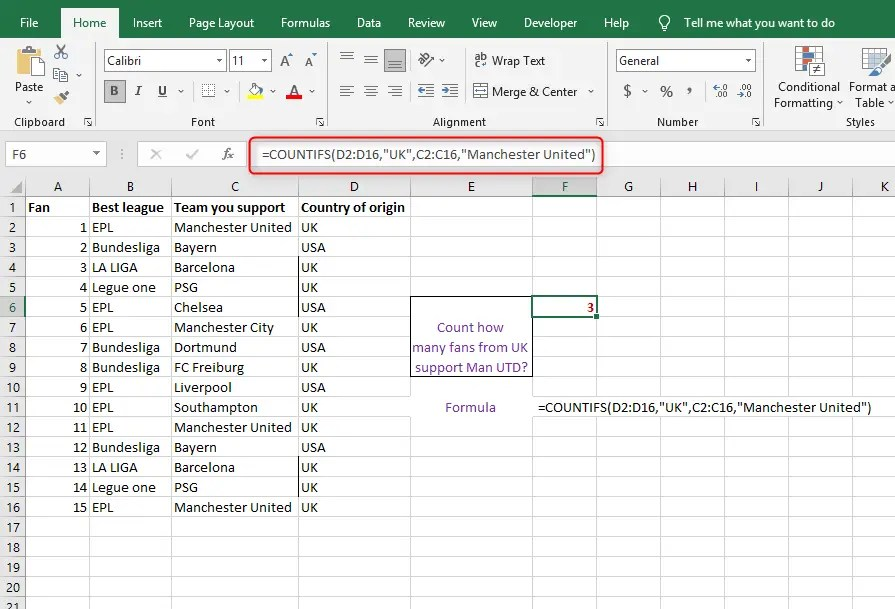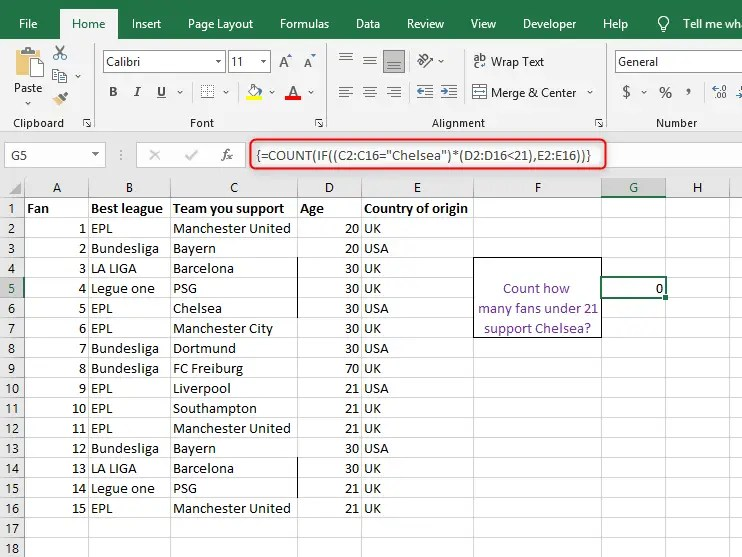# How to count per category in excel

While doing data analysis, you will one way need the skill to count data per category. This skill is important when you want to tally data by category. Excel provides you with multiple ways that you can use to count cells in rows or columns of data by category. The article will provide you with a comprehensive summary of methods that will interactively show you the way to do it. Here we go.

## Count per category using worksheet functions

You can use worksheet functions to count the number of cells in a range that satisfies a specific category’s condition (also known as criteria). You simply need to do is specify the condition using a worksheet function.

For instance, you want to count the number of times a given text or number appears in a range of cells. Then the CountIF, COUNT IFS, and a combination of IF and COUNT functions will be of great use.

### 1. Counting per category in a range using the COUNTIF function

You can be able to use the COUNTIF function whenever you want to count how many times a given value appears in a range of cells. The function counts how many times a given value appears in a range of cells. Here is the formula

`=COUNTIF(range, criteria)`

Whereby

Range: Range stands for the cells you wish to count

Criteria: This one stands for the cells that are about to be counted

For example, if you want to find out how many times English Premier League (EPL) appears recorded by many fans across the world as the best league. In a range containing several leagues across the world. Here is what your formula would look like

=COUNTIF(A3:A12,”EPL”)

OR

=COUNTIF(A3:A12,A3)

You will get the results figure as 4Note: COUNTIF can be used when you want to count cells that contain text, numbers, and dates. Also, the criteria used in COUNTIF supports wildcards (*,?) and logical operators (>,<,<>,=) for partial matching. For example, you can count cells with category in a range above or equal to 1000, i.e., =COUNTIF(B18:B21,”>=”&1000).### 2. Counting specific categories in a range using the COUNTIFS function

This function is just like the COUNTIF function but with a little difference. The exception is that the COUNTIFS function applies to criteria to cells across multiple ranges and counts when all of these criteria have been met. Here is the formula

COUNTIFS(criteria_range1, criteria1, [criteria_range2, criteria2]…)

Whereby

Criteria_range1: This is the first range of cells to find out the associated criteria. (Required)

Criteria 1: The criteria in the form of expression, number, text, or cell reference that define the cells to be counted, i.e., “oranges,” “80”, “>80”, C5, 80, etc. (Required).

Criteria_range2, criteria2,..: Several added ranges and their associated criteria. (Optional)

For example, if you want to find out how many fans from the UK support Manchester United. In a range containing several leagues across the world, teams, and countries of origin. Here is what your formula would look like

=COUNTIFS(D2:D16,”UK”,C2:C16,”Manchester United”)

You will get the results figure as 3Note: The function can be applied up to a category that meets 127 range/ criteria

### 3. Counting specific categories in a range using both the COUNT and IF function

You will use these two functions when you want to test a condition, and then if the results of testing the condition are true, then you will have to count the items that satisfy the category. Where the IF function tests the condition, and if the condition is true, the COUNT function counts these cells.

For example, if you wish to find out how many Under 21 fans support Chelsea. In a range containing several leagues across the world, teams, age of fans, and country of origin. Here is what your formula would look like

{=COUNT(IF((C2:C16=”Chelsea”)*(D2:D16<21),E2:E16))}

You will get the results figure as 0Note: The second IF argument must be a number for the formula to work. Also, press CTRL+SHIFT+ENTER to get the results since this is an array formula